login  home  contents  what's new  discussion  bug reports help  links  subscribe  changes  refresh  edit

We want to compute characters of wreath product Z_3^(Z_3)*Z_3

First, we represent the group in computer giving generators as permutations:

fricas
pG := Permutation(Integer)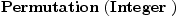(1)
Type: Type
fricas
g1 := cycle([1,2,3])$pG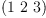(2) Type: Permutation(Integer) fricas g2 := cycle([1,4,7])$pG*cycle([2,5,8])$pG*cycle([3,6,9])$pG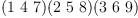(3)
Type: Permutation(Integer)
fricas
gens := [1$pG, g1, g2]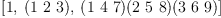(4) Type: List(Permutation(Integer)) Check the order: fricas order(permutationGroup(gens))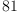(5) Type: PositiveInteger? Now, we compute list of elements. We will repeatedly multiply pending list of elements by generators. Due to length we do no print results. fricas mul_sets(s1, s2) == l := [[g1*g2 for g1 in s1] for g2 in s2] removeDuplicates(reduce(append, l)) Type: Void fricas ls := gens; Type: List(Permutation(Integer)) fricas for i in 1..20 repeat ls := mul_sets(ls, gens) fricas Compiling function mul_sets with type (List(Permutation(Integer)), List(Permutation(Integer))) -> List(Permutation(Integer)) Type: Void Check that we obtained all elements: fricas #ls(6) Type: PositiveInteger? Now, we want to compute conjugacy classes. rl below is list of remaining elements to process. fricas classes : List(List(Permutation(Integer))) := []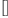(7) Type: List(List(Permutation(Integer))) fricas rl := ls; Type: List(Permutation(Integer)) fricas while not(empty?(rl)) repeat cl := removeDuplicates([g^(-1)*rl(1)*g for g in ls]) classes := cons(cl, classes) rl := setDifference(rl, cl) Type: Void fricas -- reverse for more natural order classes := reverse!(classes); Type: List(List(Permutation(Integer))) fricas -- check for correct number of classes #classes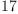(8) Type: PositiveInteger? Now we want to compute multiplication table for classes. fricas -- Auxiliary function to multiply element g1 by class cl1. -- Input: -- g1 - element -- cl1 - class -- lcl - list of all classes product_of_classes(g1, cl1, lcl) == res := []$List(Integer)
els := [g1*g2 for g2 in cl1]
for cl2 in lcl repeat
sum := 0$Integer for g2 in els repeat if member?(g2, cl2) then sum := sum + 1 res := cons(sum, res) reverse!(res) Type: Void fricas -- Auxiliary function to multiply class cl1 by class cl2 -- Input: -- cl1 and cl2 - classes -- lcl - list of all classes -- ncll - list of cardinalities of classes in lcl product_of_classes2(cl1, cl2, lcl, nlcl) == res1 : List(Integer) := product_of_classes(cl1(1), cl2, lcl) n := #cl1 [((k*n) exquo l)::Integer for k in res1 for l in nlcl] Type: Void fricas -- remember cardinalities of classes for future use nlcl := [#cl for cl in classes]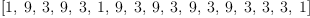(9) Type: List(NonNegativeInteger?) fricas -- empty multiplication table mt := []$List(Matrix(Integer))(10)
Type: List(Matrix(Integer))
fricas
-- Fill the multiplication table
for i in 1.. for cl1 in classes repeat
mti := new(17, 17, 0)$Matrix(Integer) for j in 1.. for cl2 in classes repeat pl := product_of_classes2(cl1, cl2, classes, nlcl) for k in 1.. for coeff in pl repeat mti(k, j) := coeff mt := cons(mti, mt) fricas Compiling function product_of_classes with type (Permutation(Integer ), List(Permutation(Integer)), List(List(Permutation(Integer)))) -> List(Integer) fricas Compiling function product_of_classes2 with type (List(Permutation( Integer)), List(Permutation(Integer)), List(List(Permutation( Integer))), List(NonNegativeInteger)) -> List(Integer) Type: Void fricas mt := reverse!(mt); Type: List(Matrix(Integer)) Computation of characters will be done over finite field, in particular for p = 1459 we have all needed roots of unity. We will produce matrix of multiplication by random element and compute its eigenvectors. fricas pF := PrimeField(1459)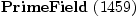(11) Type: Type fricas A := reduce(_+, [(random()$pF)*m::Matrix(pF) for m in mt]);
Type: Matrix(PrimeField?(1459))
fricas
-- check if eigenvalues are distinct
#eigenvalues(A)(12)
Type: PositiveInteger?
fricas
-- list of eigenvectors
le1 := [eir.eigvec for eir in eigenvectors(A)];
Type: List(List(Matrix(Fraction(Polynomial(PrimeField?(1459))))))
fricas
-- convert to right type
le := [column(first(ev)::Matrix(PrimeField(1459)), 1) for ev in le1];
Type: List(Vector(PrimeField?(1459)))

By normalizing and pass from g to g^(-1) we get characters from eigenvectors

fricas
-- Compute table of inverses for classes
inv := new(17, 0)$Vector(Integer); Type: Vector(Integer) fricas for i in 1.. for cl1 in classes repeat g1 := first(cl1)^(-1) for j in 1.. for cl2 in classes repeat if member?(g1, cl2) then inv(i) := j break Type: Void fricas -- Auxiliary function to compute scalar product <f, f> of central function -- f with itself. Input -- f - function as vector of values corresponding to classes -- inv - table of inverses for classes -- nlcl - list of cardinalities of classes skalar_product(f, inv, nlcl) == n : Integer := 0 s : pF := 0 for i in 1..17 for k in nlcl repeat n := n + k s := s + f(i)*f(inv(i))*(k::pF) s/(n::pF) Type: Void fricas -- Auxiliary function to normalize eigenvectors so that <f, f> = 1 and -- value at e is positive. Input -- le - list of eigenvectors -- inv - table of inverses -- nlcl - list of cardinalities of classes normalize_vectors(le, inv, nlcl) == res := []$List(Vector(pF))
il := parts(inv)
-- needed for computation of roots
g := primitiveElement()$pF for ev in le repeat sp := skalar_product(ev, inv, nlcl) k1 := discreteLog(sp) odd?(k1) => error "sp always is a square" a1 := g^(-(k1 quo 2)) n := ev(1)*a1 -- We choose root so that value at e is positive, that is less than p/2. Since values are much -- smaller than that we can use 700 in test below. if (n::Integer) > 700 then a1 := -a1 ev1 := ev*a1 res := cons(vector([ev1(k) for k in il])$Vector(pF), res)
reverse!(res)
Type: Void

Here we get list of characters

fricas
)set output tex off

fricas
)set output algebra on
lch := normalize_vectors(le, inv, nlcl);
fricas
Compiling function skalar_product with type (Vector(PrimeField(1459)
), Vector(Integer), List(NonNegativeInteger)) -> PrimeField(1459)
fricas
Compiling function normalize_vectors with type (List(Vector(
PrimeField(1459))), Vector(Integer), List(NonNegativeInteger))
-> List(Vector(PrimeField(1459)))
Type: List(Vector(PrimeField?(1459)))
fricas
lch
(31)
[[3, 0, 0, 0, 0, 3, 0, 0, 0, 0, 0, 0, 0, 439, 0, 1017, 3],
[1, 339, 339, 1, 1119, 1, 1119, 1119, 1119, 339, 1, 339, 339, 1, 1119, 1, 1]
,
[1, 1119, 1, 1119, 1, 1, 1119, 1, 339, 1, 339, 1, 339, 1, 1, 1, 1],
[3, 0, 679, 0, 780, 1017, 0, 341, 0, 1118, 0, 1121, 0, 0, 338, 0, 439],
[1, 1, 1119, 339, 339, 1, 1119, 339, 1, 1119, 1119, 1119, 339, 1, 339, 1, 1]
,
[1, 1, 339, 1119, 1119, 1, 339, 1119, 1, 339, 339, 339, 1119, 1, 1119, 1, 1]
,
[1, 339, 1119, 1119, 339, 1, 1, 339, 1119, 1119, 339, 1119, 1, 1, 339, 1, 1]
,
[1, 339, 1, 339, 1, 1, 339, 1, 1119, 1, 1119, 1, 1119, 1, 1, 1, 1],
[3, 0, 1118, 0, 338, 1017, 0, 780, 0, 1121, 0, 679, 0, 0, 341, 0, 439],
[1, 1119, 1119, 1, 339, 1, 339, 339, 339, 1119, 1, 1119, 1119, 1, 339, 1, 1]
,
[1, 1119, 339, 339, 1119, 1, 1, 1119, 339, 339, 1119, 339, 1, 1, 1119, 1, 1]
,
[1, 1, 1, 1, 1, 1, 1, 1, 1, 1, 1, 1, 1, 1, 1, 1, 1],
[3, 0, 780, 0, 679, 439, 0, 1121, 0, 338, 0, 341, 0, 0, 1118, 0, 1017],
[3, 0, 0, 0, 0, 3, 0, 0, 0, 0, 0, 0, 0, 1017, 0, 439, 3],
[3, 0, 1121, 0, 341, 1017, 0, 338, 0, 679, 0, 1118, 0, 0, 780, 0, 439],
[3, 0, 341, 0, 1121, 439, 0, 1118, 0, 780, 0, 338, 0, 0, 679, 0, 1017],
[3, 0, 338, 0, 1118, 439, 0, 679, 0, 341, 0, 780, 0, 0, 1121, 0, 1017]]
Type: List(Vector(PrimeField?(1459)))
fricas
-- Check that squares of dimensions sum to correct value
reduce(_+, [ch(1)^2 for ch in lch])
(32)  81
Type: PrimeField?(1459)

Comment: Using characters over finite field we could also compute values of characters over complex numbers, but we stop here.

 Subject:   Be Bold !! ( 14 subscribers )